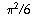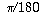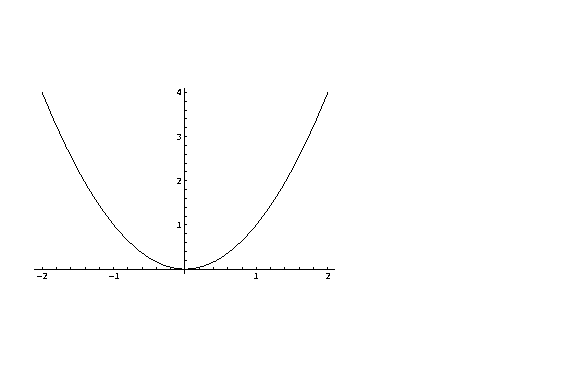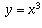Navigation Panel:(These buttons explained below)Question Corner and Discussion Area

# Infinity, Pi and Symmetry

Asked by Rudy Shearer, student, westbourne on July 3, 1997:
Please tell me about the concepts of infinity,and symmetry.
Before I begin, I will note that a truly detailed account of any of these topics could easily fill a book (I know that there is at least one book dedicated to the history ofand I have little doubt that there are entire books on symmetry as well). What follows are a few words about each topic.

For information about infinity, refer to the page Does Infinity Exist?.

Pi is, quite simply, a number. It has been known of since ancient times -- there are references to it in the Bible and in Greek literature, to name a few. Over the years we have gained more and more knowledge ofand calculated it to progressively greater and greater precisions (it is now known to millions of decimal places).

The definition ofis remarkably simple. It is the ratio between the circumference and diameter of a circle. To a few decimal places its value is 3.14159265. . . . Similar to the square root of 2, it is an irrational number and its digits never repeat. In this sense (and others) it is somewhat pathological.

Nevertheless,turns up frequently in applied mathematics problems, sometimes in surprising ways. For instance, the sum 1 + 1/4 + 1/9 + 1/16 + 1/25 +. . . converges to(you may see the proof of this in a calculus course some day).

It turns out that there is a unit of angular measure frequently used in mathematics which involves. If two lines intersect at a point, we can measure the angle between them as follows. Draw a circle (with any radius you like) centered at the point where the lines cross. Now the measure of the angle is given by the length of its arc on the circle divided by the radius of the circle. This is the measure of the angle in radians. There areradians in a full circle and we can therefore convert degrees to radians by multiplying by.

Symmetry is one of Mathematicians' greatest friends, as it often helps to simplify their lives. It is difficult to define exactly what it is, though it always refers to some sort of self-similarity of an object. This sort of repetition allows us to study a small part of an object and still have a good feel for how it behaves as a whole.

Often this symmetry is in the form of a reflection: one half of the object is the mirror image of the other half of the object. Consider the graph of the parabola. The section of the parabola to the right of the y-axis is just a reflection of the other half of the parabola.Other times symmetry occurs as reflection through a point. The graph of the curveexhibits this type of symmetry. If we wanted to find the signed area between this curve and the x-axis between the lines x = -1 and x = +1, we could note that the area to the left of the y-axis has the same value, with a change of sign, as the area to the right of the y-axis. Without performing any significant calculations we can use symmetry to deduce that the total area is 0.Geometric solids often exhibit some sort of useful symmetry. Suppose we wanted to calculate the volume of an icosahedron (a 20 sided object whose faces are all equilateral triangles). The task seems difficult if we fail to notice that our solid is simply 20 identical pyramids glued together. We can just find the volume of one of these pyramids and then find the volume of the whole icosahedron by multiplying by 20.

Symmetry can occur in more subtle ways as well. Sometimes proofs themselves exhibit some symmetry. Instead of repeating one's self, authors often prove only one of the cases of a theorem. They then argue that the other similar cases are proved in a symmetric way.

[ Submit Your Own Question ] [ Create a Discussion Topic ]

This part of the site maintained by (No Current Maintainers)
Last updated: April 19, 1999
Original Web Site Creator / Mathematical Content Developer: Philip Spencer
Current Network Coordinator and Contact Person: Joel Chan - mathnet@math.toronto.edu

Navigation Panel: